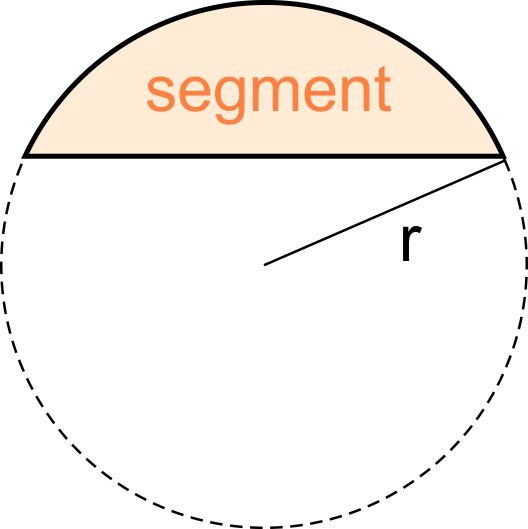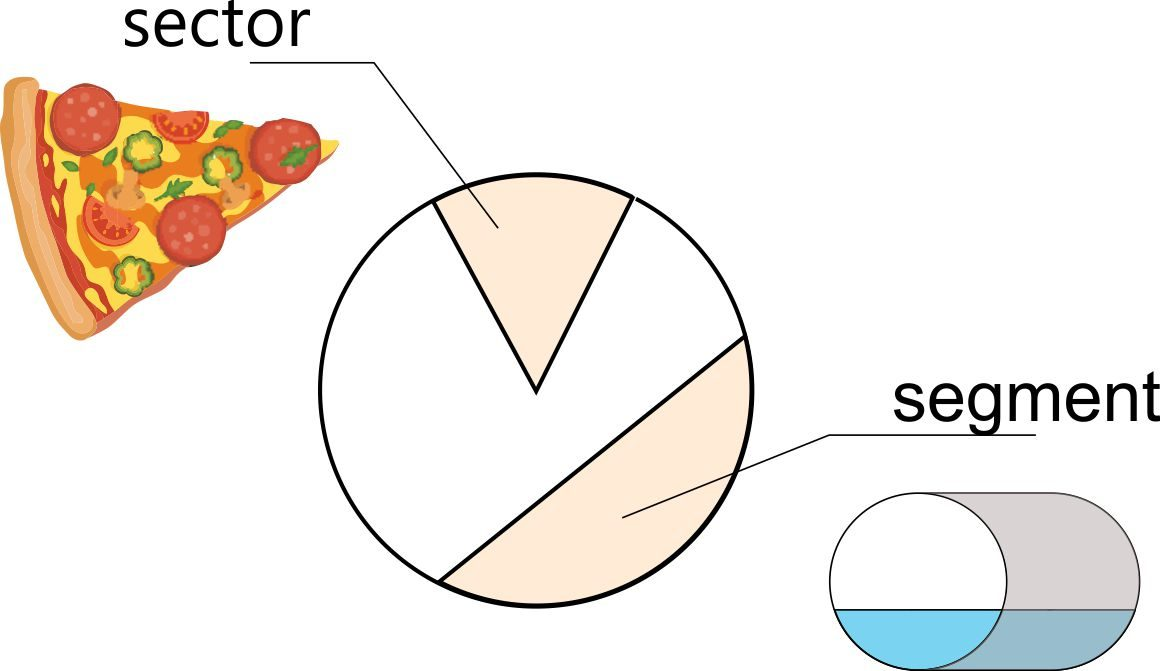# Segment Area Calculator

Created by Hanna Pamuła, PhD
Reviewed by Bogna Szyk and Jack Bowater
Last updated: Oct 08, 2022

Use this segment area calculator to quickly compute the area of a segment. It can also be used to find chord length and arc length. If you're unsure what a segment of a circle is or even what a chord of a circle is, don't feel embarrassed – just scroll down to find a few definitions and some self-explanatory images.

## What is a segment of a circle? ⌓If you want to understand what the segment of a circle is, try to imagine cutting part of a circle off with a single, straight cut. And that's it! You've just created two parts of the circle, and the smaller one is called the circular segment. A more formal mathematical definition says that:

A circular segment is a region bounded by a chord and the arc of a circle (of less than 180°)

If it's equal to 180°, then it's simply a half circle – semicircle. According to some definitions, the central angle doesn't need to be smaller than 180° – in that case, you can say that cutting a circle with a line gives you two segments: a major segment and a minor segment.Have a look at the picture below to help you visualize the difference between segment and sector, as those two names are sometimes confused:## What is a chord of a circle?A chord is a line that connects two points on a circle. The infinite line extension of a chord is called a secant. The special case of a chord is one that passes through the center of a circle – and that's the circle diameter, of course!

## Formulas for a segment of a circle area

To find the circle segment area, you need to know at least two variables. In our segment area calculator, you'll find two popular formulas implemented:1. Formula given radius and central angle

Asegment = 0.5 × r² × (α – sin(α))

Where does this formula come from? You can look at the segment area as the difference between the area of a sector and the area of an isosceles triangle formed by the two radii:

Asegment = Asector - Atriangle

Knowing the sector area formula:

An equation for the area of an isosceles triangle, given arm and angle:

You can find the final equation for the segment of a circle area:

Asegment = Asector - Aisosceles triangle = (0.5 × r² × α) - (0.5 × r² × sin(α)) = 0.5 × r² × (α – sin(α))

2. Formula given radius and height

Asegment = r² × arccos((r-h)/r) - (r-h) × √(2 × r × h - h²)

where h is the height of a segment, also known as sagitta.

This formula may be useful when you need to calculate, e.g., the volume of water in a pipe that is not completely full.

## This segment area calculator can work as a chord length calculator as well!

Let's find out how to use this segment area calculator. In our example, we want to find the area of the cross-section of a partially filled pipe:

1. Input the circle radius. Assume our pipe radius is 5 in.
2. Enter the second variable. Let's say that it's filled 3 inches high, so input that value into the height box.
3. There you go, that's it! Now we know that our segment area is equal to 19.8 in². Additionally, we determined the chord length (9.17 in), arc length (11.6 in), and central angle (132.84°).
Hanna Pamuła, PhD
inCentral angle (α)
deg
Height (h)
in
Arc length (L)
in
Chord length (c)
in
Segment area (A)
in²
People also viewed…

### Arc length

Our arc length calculator can calculate the length of an arc of a circle and the area of a sector.

### Car heat

The hot car calculator shows how fast a car's interior heats up during a summer day.

### Coffee kick

A long night of studying? Or maybe you're on a deadline? The coffee kick calculator will tell you when and how much caffeine you need to stay alert after not sleeping enough 😀☕ Check out the graph below!

### Substitution method

Substitution method calculator shows you step-by-step how to solve systems of linear equations using the substitution method.